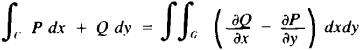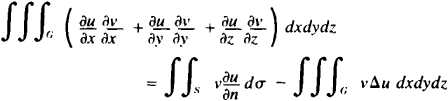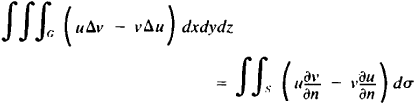# Green's Formulas

The following article is from The Great Soviet Encyclopedia (1979). It might be outdated or ideologically biased.

## Green’s Formulas

formulas of integral calculus interrelating various types of integrals. The simplest of them relates a double integral over region G to a curvilinear integral along boundary C of region G and has the formThis formula was known even to L. Euler (1771). Two other formulas first published by George Green in 1828 in connection with research on the theory of potential are(the first Green formula or the preliminary Green formula) andHere, G is the region of a three-dimensional space, surface 5 is the boundary of this region, Δu = ∂2u/∂x2 + ∂2u/∂y2 + ∂2u/∂z2v is defined in the same way) is a Laplace operator, and ∂u/∂n, ∂v ∂n are derivatives in the direction of the outer normal to S.